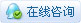3124浏览

# [项目] 基于云雀气象仪与Mind+数据面板的大屏可视化校园气象站操作简便，效果惊艳

• 离线工作方式简介名称 功能 可选项 默认 Communication 设置通信方式 I2C/UART（固定115200） I2C Sample rate 设置数据采样率，单位秒 1~43200（12小时） 1秒 Record 设置存储功能是否开启 ON/OFF OFF Radial 预留功能，勿动 Delay record 设置存储功能延时启动时间，单位秒 10~60 10秒 Light Switch 设置RGB指示灯是否开启 ON/OFF ON

• 在线工作方式详解

• 1.    线路连接• 2.    升级行空板SIoT服务器• 3.    程序设计Python代码：

``````#  -*- coding: UTF-8 -*-

# MindPlus
# Python
from DFRobot_Atmospherlum import *
from pinpong.board import Board
from unihiker import GUI
import datetime
import time
import siot

# 自定义函数
def XiaoShiFengLiZuiDaZhi():
global FengLiJiShi
global FengLiMAX
if (FengLiJiShi == (int(float(datetime.datetime.now().hour)))):
if (FengLiMAX < (int(float((yunque_i2c.get_value("speed")))))):
FengLiMAX = (int(float((yunque_i2c.get_value("speed")))))
else:
FengLiMAX = (int(float((yunque_i2c.get_value("speed")))))
FengLiJiShi = (int(float(datetime.datetime.now().hour)))

Board().begin()
u_gui=GUI()
siot.connect()
siot.loop()
yunque_i2c = DFRobot_Atmospherlum_I2C(0x42)
while (yunque_i2c.begin() != 0):
print("yunque_i2c initialize failed!!")
time.sleep(1)
print("Sensor initialize success!!")
yunque_i2c.set_local_time()
time.sleep(1)

FengXiang = {"E":"东","S":"南","W":"西","N":"北","NE":"东北","SE":"东南","SW":"西南","NW":"西北"}
FengLiJiShi = (int(float(datetime.datetime.now().hour)))
FengLiMAX = (int(float((yunque_i2c.get_value("speed")))))
UI=u_gui.draw_image(image="UI1.png",x=0,y=0)
u_gui.draw_text(text="温度：",x=28,y=20,font_size=20, color="#000000")
u_gui.draw_text(text="湿度：",x=28,y=60,font_size=20, color="#000000")
u_gui.draw_text(text="风向：",x=28,y=100,font_size=20, color="#000000")
u_gui.draw_text(text="风力：",x=28,y=140,font_size=20, color="#000000")
u_gui.draw_text(text="气压：",x=28,y=180,font_size=20, color="#000000")
TEMP=u_gui.draw_digit(text=(yunque_i2c.get_value("Temp")),x=110,y=30,font_size=20, color="#000000")
Humi=u_gui.draw_digit(text=(yunque_i2c.get_value("Humi")),x=110,y=70,font_size=20, color="#000000")
Direction=u_gui.draw_text(text=(yunque_i2c.get_value("dir")),x=110,y=100,font_size=20, color="#000000")
Speed=u_gui.draw_digit(text=(yunque_i2c.get_value("speed")),x=110,y=150,font_size=20, color="#000000")
Pressure=u_gui.draw_digit(text=(yunque_i2c.get_value("Pressure")),x=110,y=190,font_size=20, color="#000000")

while True:
XiaoShiFengLiZuiDaZhi()
TEMP.config(text=(yunque_i2c.get_value("Temp")))
siot.publish_save(topic="siot/温度", data=(yunque_i2c.get_value("Temp")))
Humi.config(text=(yunque_i2c.get_value("Humi")))
siot.publish_save(topic="siot/湿度", data=(yunque_i2c.get_value("Humi")))
Direction.config(text=(FengXiang[(yunque_i2c.get_value("dir"))]))
siot.publish_save(topic="siot/风向", data=(FengXiang[(yunque_i2c.get_value("dir"))]))
Speed.config(text=FengLiMAX)
siot.publish_save(topic="siot/风力", data=(yunque_i2c.get_value("speed")))
siot.publish_save(topic="siot/小时风力最大值", data=FengLiMAX)
Pressure.config(text=(yunque_i2c.get_value("Pressure")))
siot.publish_save(topic="siot/气压", data=(yunque_i2c.get_value("Pressure")))
time.sleep(10)• 4.    定制数据面板Mind+数据面板实质上是一个独立的模块，并不与当前编辑的程序关联。你甚至可以在打开A项目程序的状态下，进行配套B项目的数据面板编辑。• 5.    测试与部署项目小结

1.为新课标的落地提供器材支撑

2.从“观”的浅层步入“做”的深层

3.创设独到的数字环境育人价值2022版新课标背景下，DF对云雀气象仪、Mind+数据面板的研发，以及我和PCDC小研究员们在这个项目上的探索都只不过是信息科技跨学科主题方向的微小努力。虽是粗浅的尝试，但却让我们有了更多的期待与确信！### tangjie初级技师

 v1.8版本的mind+在哪下载？### 腿毛利小五郎中级技师

 不错！！！

 厉害厉害，不错不错### Lb0sihNKI9NJ学徒

 厉害，感觉很有意思### digi_cow高级技匠 楼主|

 tangjie 发表于 2023-4-4 11:44 v1.8版本的mind+在哪下载？ 目前还处于内测阶段

 6666666666666

 学习学习！您需要登录后才可以回帖 登录 | 立即注册 本版积分规则 回帖并转播 回帖后跳转到最后一页